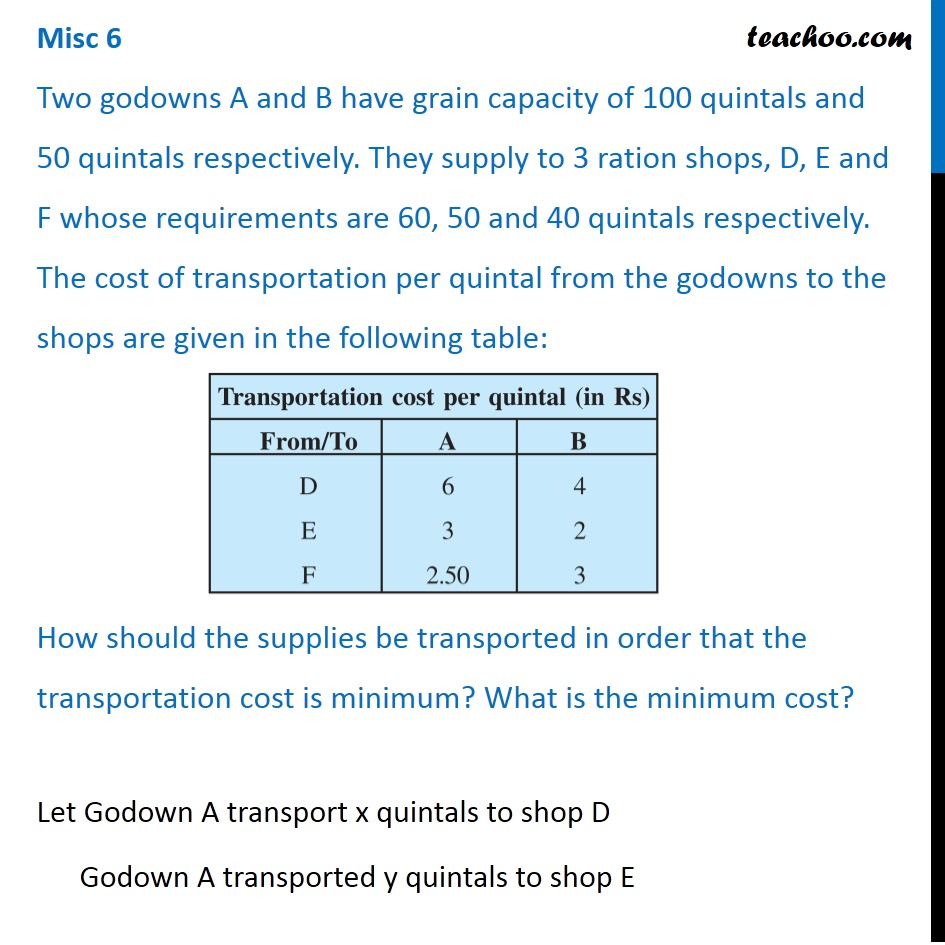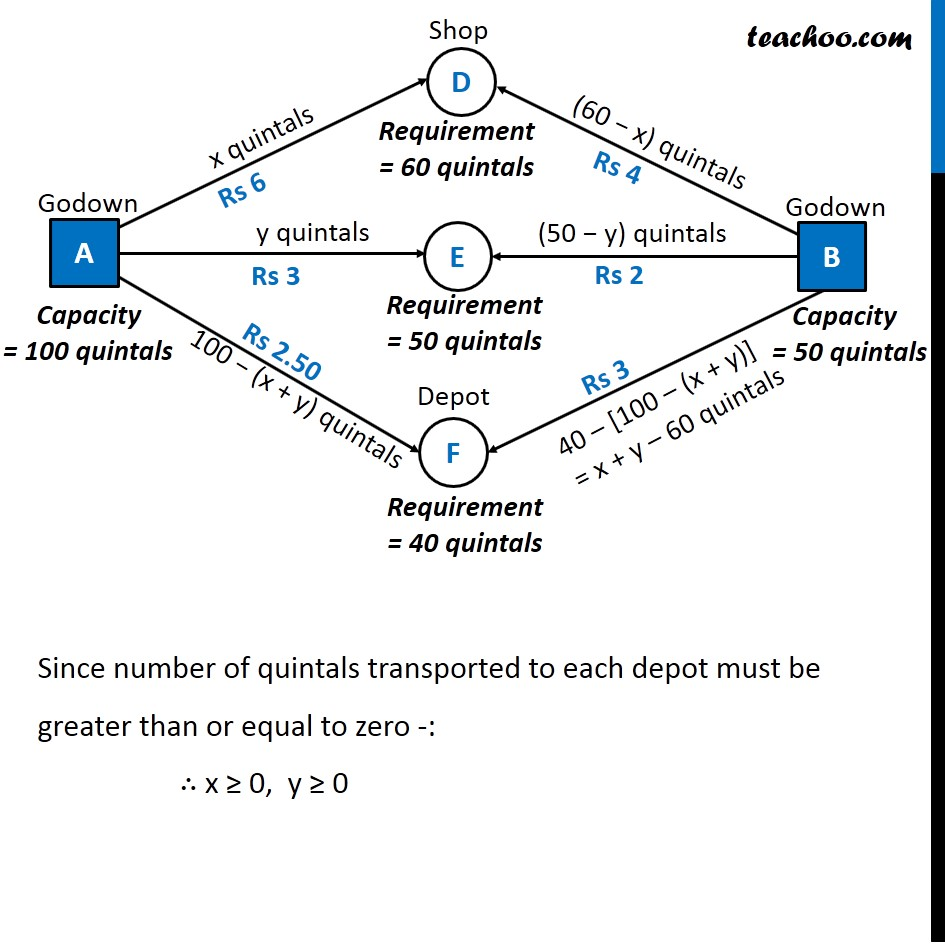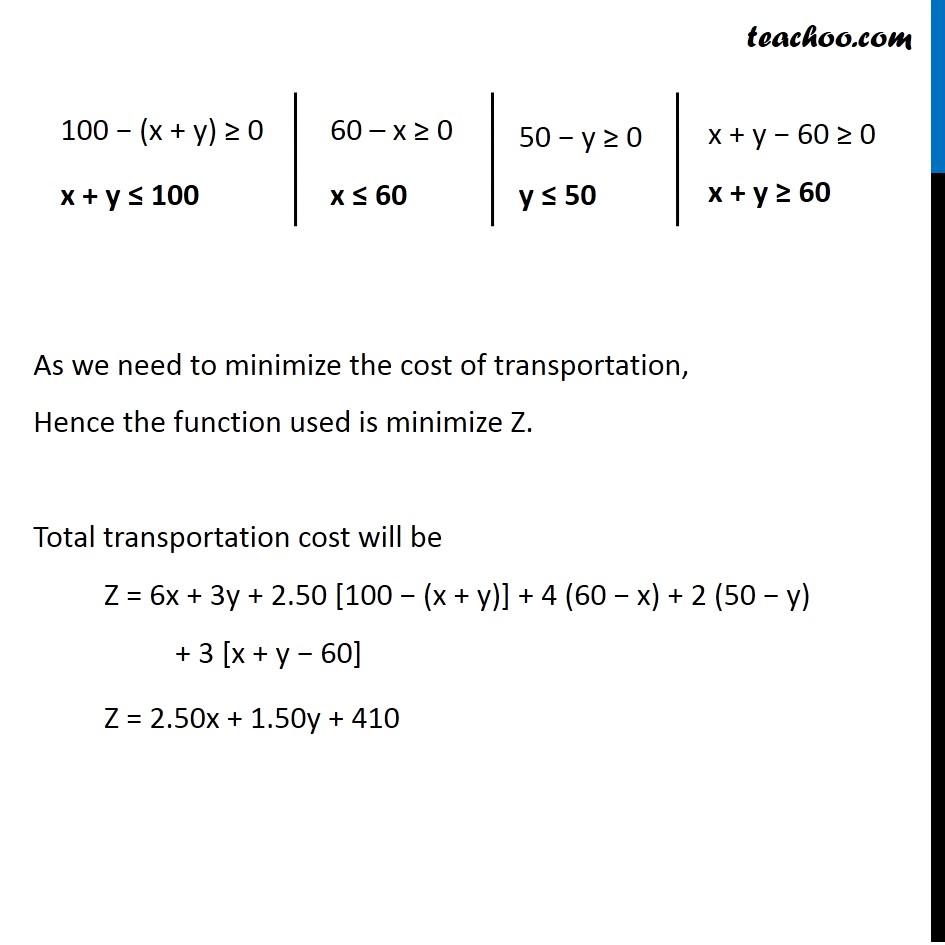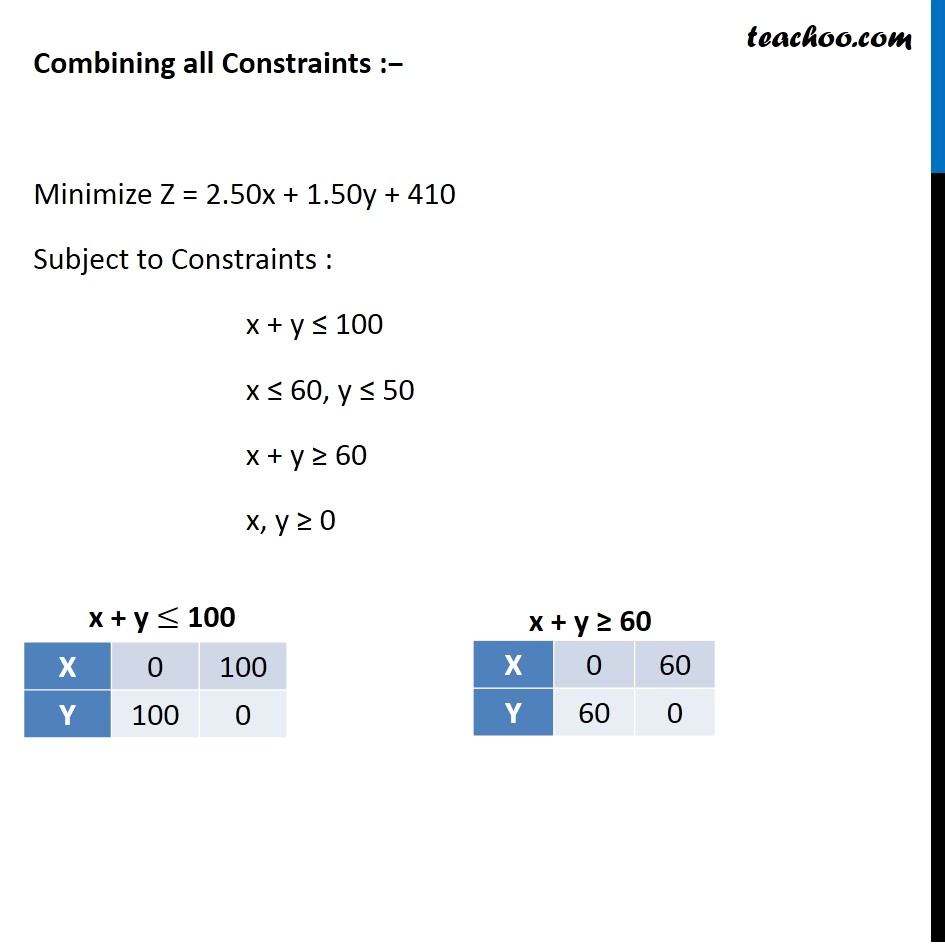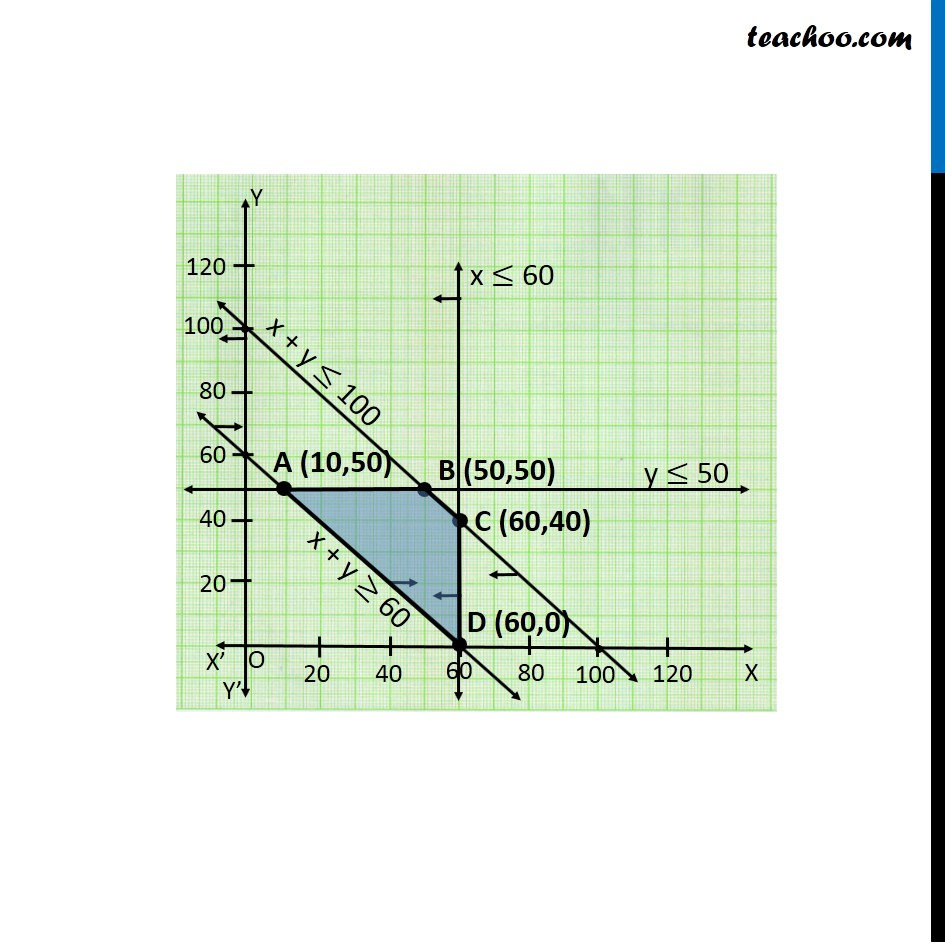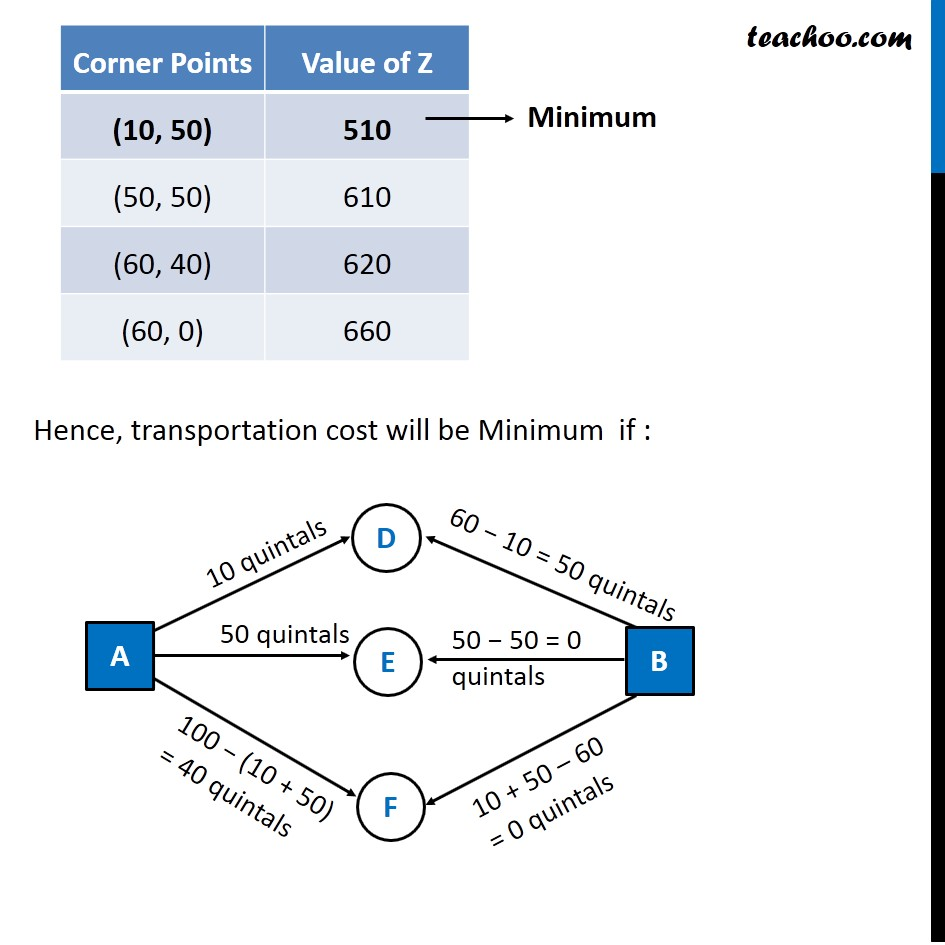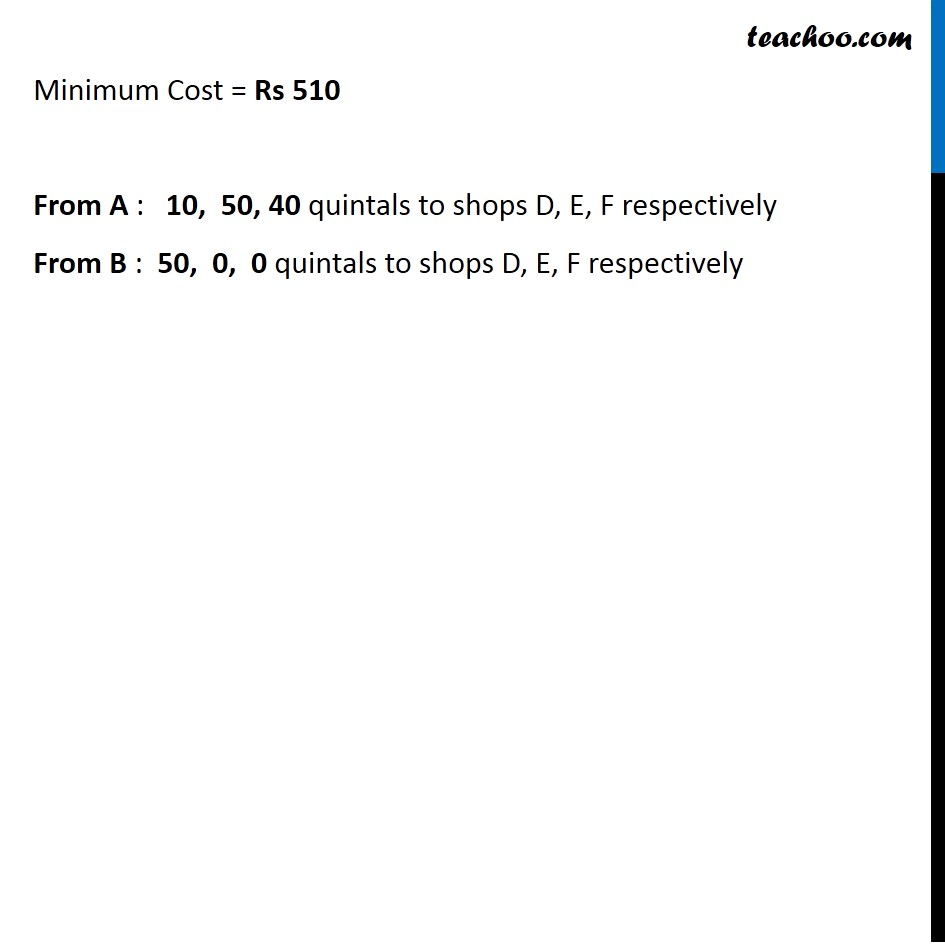1. Chapter 12 Class 12 Linear Programming (Term 1)
2. Serial order wise
3. Miscellaneous

Transcript

Misc 6 Two godowns A and B have grain capacity of 100 quintals and 50 quintals respectively. They supply to 3 ration shops, D, E and F whose requirements are 60, 50 and 40 quintals respectively. The cost of transportation per quintal from the godowns to the shops are given in the following table: How should the supplies be transported in order that the transportation cost is minimum? What is the minimum cost? Let Godown A transport x quintals to shop D Godown A transported y quintals to shop E Since number of quintals transported to each depot must be greater than or equal to zero -: ∴ x ≥ 0, y ≥ 0 100 − (x + y) ≥ 0 x + y ≤ 100 60 – x ≥ 0 x ≤ 60 50 − y ≥ 0 y ≤ 50 x + y − 60 ≥ 0 x + y ≥ 60 As we need to minimize the cost of transportation, Hence the function used is minimize Z. Total transportation cost will be Z = 6x + 3y + 2.50 [100 − (x + y)] + 4 (60 − x) + 2 (50 − y) + 3 [x + y − 60] Z = 2.50x + 1.50y + 410 Combining all Constraints :− Minimize Z = 2.50x + 1.50y + 410 Subject to Constraints : x + y ≤ 100 x ≤ 60, y ≤ 50 x + y ≥ 60 x, y ≥ 0 Hence, transportation cost will be Minimum if : Hence, transportation cost will be Minimum if : Minimum Cost = Rs 510 From A : 10, 50, 40 quintals to shops D, E, F respectively From B : 50, 0, 0 quintals to shops D, E, F respectively

Miscellaneous

Chapter 12 Class 12 Linear Programming (Term 1)
Serial order wise